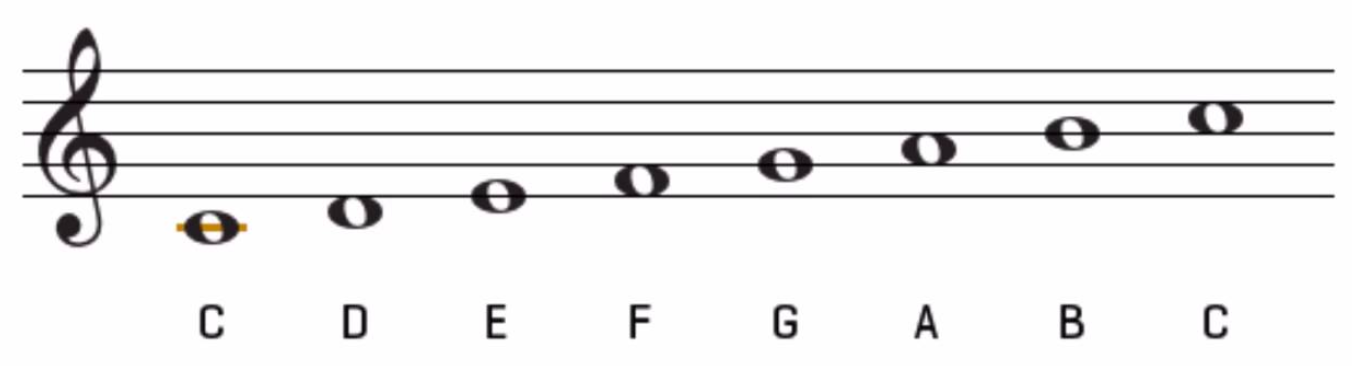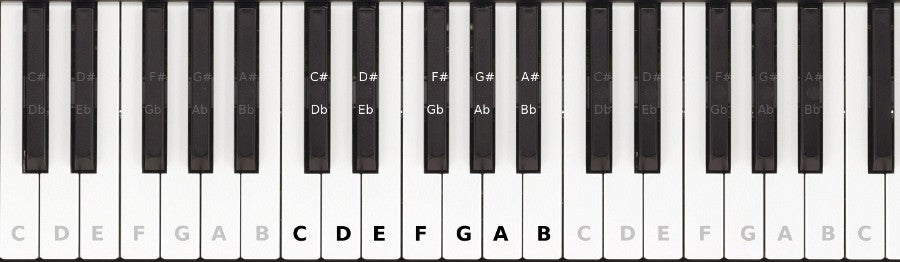# The Major Scale

The Major Scale

Remember, if you have any trouble understanding any of this, please send me an email. I will find another way of wording it, or I'll find an image, or whatever I need to do to make sure you understand. Thanks! dfurda@hesk6.us

This week, let's see how the major scale (Do, Re, Mi, Fa, Sol, La, Ti, Do) gives us Key Signatures (which sharps or flats to use).

The Major Scale-As always in music, we start with the key of C. When you press each white key on the piano going from one C to the next C, we hear the Major Scale. (This is why there are no sharps or flats in the key of C major.)

To understand this more, we take a look at the interval (distance) between the notes.On the piano keyboard, when you go from one note to the very next note (whether black or white), we call that a half step. So, it would make sense that if we go up to the next note from there, we have now gone a whole step. For example, going from C to C# is only a half step, there is nothing in-between. But going from C to D is a whole step because there is a key in-between (C#/D-flat).

Going back to the C Major Scale, the step pattern of the notes going up is...
C- whole- D- whole- E- half- F- whole- G- whole- A- whole- B- half- C

So, for any Major Scale, the step pattern ascending (going up) is...
Whole, whole, half, whole, whole, whole, half, which is abbreviated to (W=whole, H=half)

W W H W W W H

Let's see how this pattern helps us figure out Key Signatures.Figuring out Key Signatures using the Major Scale-
We already know from the top of the page, that C Major has no sharps and no flats.

Let's take a look at D Major.
Obviously, we start with D, then we need to use the WWHWWWH pattern going up the keyboard.

D up a WHOLE step is E (because there is a black key in-between), E up a WHOLE step is F# (because F is in-between), F# up a HALF step is G (because there is nothing in-between), G up a WHOLE step is A (because there is a black key in-between), A up a WHOLE step is B (because there is a black key in-between), B up a WHOLE step is C# (because C is in-between), C# up a HALF step is D (because there is nothing in-between). So the D Major Scale is...

D   E   F#  G   A   B   C#  D

So, we have two sharps in the key of D Major.

Let's do one more together... E Major.

E up a WHOLE step is F# (because F is in-between), F# up a WHOLE step is G# (because G is in-between), G# up a HALF step is A (because there is nothing in-between), A up a WHOLE step is B (because there is a black key in-between), B up a WHOLE step is C# (because C is in-between), C# up a WHOLE step is D# (because D is in-between), D# up a HALF step is E because there is nothing in-between). So the E Major Scale is...

E   F#  G#  A   B   C#  D#  E

So, we have four sharps in the key of E Major.

This is as far as you need to go this week. You may look at what is below, but I decided it was becoming too complicated all at once. I just don't want to lose the work I did already. You'll probably do this part next week.This is where the Circle of Fifth (image below) comes into play. Although, you can use the above pattern from any note and work out the Key Signature, we usually go in order by going up a fifth (an interval) at a time.

To count up a fifth, the note we start on is 1, going up we count each letter, then the note we're going to gives up the interval.

Here's an example...

We start with C, so C is 1. Then, D is 2, E, is 3, F is 4, and G is 5. So, a fifth above C is G.

Another example...

Let's start with G. G= 1, A= 2, B= 3, C= 4, D= 5, so a fifth above G is D.

Last example, for now...

Let's find the fifth above D. D= 1, E= 2, F= 3, G= 4, A= 5, so a fifth above D is A.